# Feature Selection TechniquesVariable selection is used to identify a subset of the available inputs that accurately predict and output.
Why need variable selection?

With a smaller dataset, you can reduce the cost for data collection/cleaning, improve speed/performance by decreasing computation time and scoring efforts. And also improve interchangeability, remove multicollinearity & irralational coefficients, treat missing data, reduce redundancy. With too many variables, it will destabilize the parameter estimates and increase the risk of overfiting and noise.

Things to consider before variable selection:

Decide how you intend to use your model

• Describe the relationship between variables
• Which predictors are statistically significant
• Model has reasonable goodness-of-fit
• Ability to predict
• Identify outliers and influential points – maybe exclude them at least temporily
• Add in any transformations of the variables that seem appropriate.
• Impute missing values

Variable selection techniques:

• Regression based
• Criterion based/Variable Screening: variable ranking, correlations,Variable selection based on correlation with target variable, IV, WOE
 Information Value Predictive Power < 0.02 useless for prediction 0.02 to 0.1 Weak predictor 0.1 to 0.3 Medium predictor 0.3 to 0.5 Strong predictor >0.5 Suspicious or too good to be true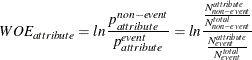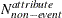: the number of non-event records that exhibit the attribute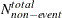: the total number of non-event records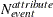: the number of event records that exhibit the attribute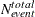: the total number of event records

• To avoid an undefined WOE, an adjustment factor,, is used: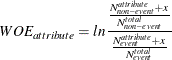You can use the WOEADJUST= option to specify a value between [0, 1] for. By default,is 0.5.

The information value (IV) is a weighted sum of the WOE of the characteristic’s attributes. The weight is the difference between the conditional probability of an attribute given an event and the conditional probability of that attribute given a non-event. In the following formula of IV,is the number of bins of a variable: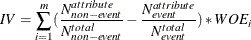• Variable Clustering
• Variable combination: principle components uncorrelated linear combinations of all input variables
• All possible: best subset. Best subsets estimates one regression model for all possible combinations of the predictor variables and chooses the best model among them.
• Automatic: stepwise, backward, forward. Stepwise selection considers adding and deleting predictors at each step of the process. Forward selection begins with a simple regression model and adds, one at a time, However, once a predictor is in the equation, it is never deleted.Backward selection begins with the model including all possible predictors and deletes, one at a time. Once a variable is deleted, it is never reconsidered for inclusion.

## Random Forest

Random forest feature importance

Random forests are among the most popular machine learning methods thanks to their relatively good accuracy, robustness and ease of use. They also provide two straightforward methods for feature selection: mean decrease impurity and mean decrease accuracy. But they come with their own gotchas, especially when data interpretation is concerned. With correlated features, strong features can end up with low scores and the method can be biased towards variables with many categories. As long as the gotchas are kept in mind, there really is no reason not to try them out on your data.

Mean decrease impurity

Random forest consists of a number of decision trees. Every node in the decision trees is a condition on a single feature, designed to split the dataset into two so that similar response values end up in the same set. The measure based on which the (locally) optimal condition is chosen is called impurity. For classification, it is typically either Gini impurity or information gain/entropy and for regression trees it is variance. Thus when training a tree, it can be computed how much each feature decreases the weighted impurity in a tree. For a forest, the impurity decrease from each feature can be averaged and the features are ranked according to this measure.

This is the feature importance measure exposed in sklearn’s Random Forest implementations (random forest classifier and random forest regressor).

 1 2 3 4 5 6 7 8 9 10 11 12 13 from sklearn.datasets import load_boston from sklearn.ensemble import RandomForestRegressor import numpy as np #Load boston housing dataset as an example boston = load_boston() X = boston["data"] Y = boston["target"] names = boston["feature_names"] rf = RandomForestRegressor() rf.fit(X, Y) print "Features sorted by their score:" print sorted(zip(map(lambda x: round(x, 4), rf.feature_importances_), names),               reverse=True)

 Features sorted by their score: [(0.5298, 'LSTAT'), (0.4116, 'RM'), (0.0252, 'DIS'), (0.0172, 'CRIM'), (0.0065, 'NOX'), (0.0035, 'PTRATIO'), (0.0021, 'TAX'), (0.0017, 'AGE'), (0.0012, 'B'), (0.0008, 'INDUS'), (0.0004, 'RAD'), (0.0001, 'CHAS'), (0.0, 'ZN')] 

There are a few things to keep in mind when using the impurity based ranking. Firstly, feature selection based on impurity reduction is biased towards preferring variables with more categories (see Bias in random forest variable importance measures). Secondly, when the dataset has two (or more) correlated features, then from the point of view of the model, any of these correlated features can be used as the predictor, with no concrete preference of one over the others. But once one of them is used, the importance of others is significantly reduced since effectively the impurity they can remove is already removed by the first feature. As a consequence, they will have a lower reported importance. This is not an issue when we want to use feature selection to reduce overfitting, since it makes sense to remove features that are mostly duplicated by other features. But when interpreting the data, it can lead to the incorrect conclusion that one of the variables is a strong predictor while the others in the same group are unimportant, while actually they are very close in terms of their relationship with the response variable.

The effect of this phenomenon is somewhat reduced thanks to random selection of features at each node creation, but in general the effect is not removed completely. In the following example, we have three correlated variables X0,X1,X2, and no noise in the data, with the output variable simply being the sum of the three features:

 1 2 3 4 5 6 7 8 9 10 11 12 13 size = 10000 np.random.seed(seed=10) X_seed = np.random.normal(0, 1, size) X0 = X_seed + np.random.normal(0, .1, size) X1 = X_seed + np.random.normal(0, .1, size) X2 = X_seed + np.random.normal(0, .1, size) X = np.array([X0, X1, X2]).T Y = X0 + X1 + X2   rf = RandomForestRegressor(n_estimators=20, max_features=2) rf.fit(X, Y); print "Scores for X0, X1, X2:", map(lambda x:round (x,3),                                     rf.feature_importances_)

 Scores for X0, X1, X2: [0.278, 0.66, 0.062] 

When we compute the feature importances, we see that X1 is computed to have over 10x higher importance than X2, while their “true” importance is very similar. This happens despite the fact that the data is noiseless, we use 20 trees, random selection of features (at each split, only two of the three features are considered) and a sufficiently large dataset.

One thing to point out though is that the difficulty of interpreting the importance/ranking of correlated variables is not random forest specific, but applies to most model based feature selection methods.

Mean decrease accuracy

Another popular feature selection method is to directly measure the impact of each feature on accuracy of the model. The general idea is to permute the values of each feature and measure how much the permutation decreases the accuracy of the model. Clearly, for unimportant variables, the permutation should have little to no effect on model accuracy, while permuting important variables should significantly decrease it.

This method is not directly exposed in sklearn, but it is straightforward to implement it. Continuing from the previous example of ranking the features in the Boston housing dataset:

 1 2 3 4 5 6 7 8 9 10 11 12 13 14 15 16 17 18 19 20 21 22 23 24 from sklearn.cross_validation import ShuffleSplit from sklearn.metrics import r2_score from collections import defaultdict X = boston["data"] Y = boston["target"] rf = RandomForestRegressor() scores = defaultdict(list) #crossvalidate the scores on a number of different random splits of the data for train_idx, test_idx in ShuffleSplit(len(X), 100, .3):     X_train, X_test = X[train_idx], X[test_idx]     Y_train, Y_test = Y[train_idx], Y[test_idx]     r = rf.fit(X_train, Y_train)     acc = r2_score(Y_test, rf.predict(X_test))     for i in range(X.shape):         X_t = X_test.copy()         np.random.shuffle(X_t[:, i])         shuff_acc = r2_score(Y_test, rf.predict(X_t))         scores[names[i]].append((acc-shuff_acc)/acc) print "Features sorted by their score:" print sorted([(round(np.mean(score), 4), feat) for               feat, score in scores.items()], reverse=True)

 Features sorted by their score: [(0.7276, 'LSTAT'), (0.5675, 'RM'), (0.0867, 'DIS'), (0.0407, 'NOX'), (0.0351, 'CRIM'), (0.0233, 'PTRATIO'), (0.0168, 'TAX'), (0.0122, 'AGE'), (0.005, 'B'), (0.0048, 'INDUS'), (0.0043, 'RAD'), (0.0004, 'ZN'), (0.0001, 'CHAS')] 

In this example LSTAT and RM are two features that strongly impact model performance: permuting them decreases model performance by ~73% and ~57% respectively. Keep in mind though that these measurements are made only after the model has been trained (and is depending) on all of these features. This doesn’t mean that if we train the model without one these feature, the model performance will drop by that amount, since other, correlated features can be used instead.

Introducing Amazon AWS H2O package, using random forest for feature selection where missing and outliers are automatically treated.